# JP2004096557A - Image processor and image processing method - Google Patents

## Info

Publication number
JP2004096557A
JP2004096557A JP2002256841A JP2002256841A JP2004096557A JP 2004096557 A JP2004096557 A JP 2004096557A JP 2002256841 A JP2002256841 A JP 2002256841A JP 2002256841 A JP2002256841 A JP 2002256841A JP 2004096557 A JP2004096557 A JP 2004096557A
Authority
JP
Japan
Prior art keywords
shape data
frame
image
object
data
Prior art date
Legal status (The legal status is an assumption and is not a legal conclusion. Google has not performed a legal analysis and makes no representation as to the accuracy of the status listed.)
Withdrawn
Application number
JP2002256841A
Other languages
Japanese (ja)
Inventor
Osamu Itokawa

Original Assignee
Canon Inc
キヤノン株式会社
Priority date (The priority date is an assumption and is not a legal conclusion. Google has not performed a legal analysis and makes no representation as to the accuracy of the date listed.)
Filing date
Publication date
Application filed by Canon Inc, キヤノン株式会社 filed Critical Canon Inc
Priority to JP2002256841A priority Critical patent/JP2004096557A/en
Publication of JP2004096557A publication Critical patent/JP2004096557A/en
Withdrawn legal-status Critical Current

• 238000003672 processing method Methods 0.000 title claims abstract description 19
• 230000002159 abnormal effects Effects 0.000 claims abstract description 62
• 238000000034 methods Methods 0.000 claims description 44
• 230000000875 corresponding Effects 0.000 claims description 42
• 238000000605 extraction Methods 0.000 claims description 17
• 230000001131 transforming Effects 0.000 claims description 10
• 230000000694 effects Effects 0.000 description 1
• 238000005516 engineering processes Methods 0.000 description 1

## Images

•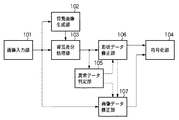•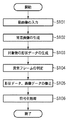•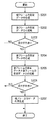•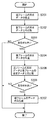••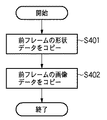•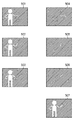•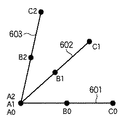•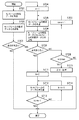•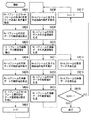•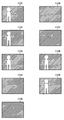•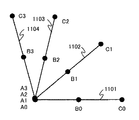•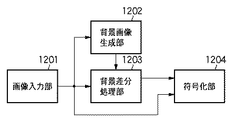•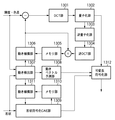•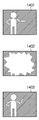•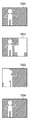## Classifications

• GPHYSICS
• G06COMPUTING; CALCULATING; COUNTING
• G06TIMAGE DATA PROCESSING OR GENERATION, IN GENERAL
• G06T9/00Image coding
• G06T9/001Model-based coding, e.g. wire frame
• GPHYSICS
• G06COMPUTING; CALCULATING; COUNTING
• G06TIMAGE DATA PROCESSING OR GENERATION, IN GENERAL
• G06T7/00Image analysis
• G06T7/20Analysis of motion
• G06T7/254Analysis of motion involving subtraction of images
• GPHYSICS
• G06COMPUTING; CALCULATING; COUNTING
• G06TIMAGE DATA PROCESSING OR GENERATION, IN GENERAL
• G06T9/00Image coding
• G06T9/20Contour coding, e.g. using detection of edges
• HELECTRICITY
• H04ELECTRIC COMMUNICATION TECHNIQUE
• H04NPICTORIAL COMMUNICATION, e.g. TELEVISION
• H04N19/00Methods or arrangements for coding, decoding, compressing or decompressing digital video signals
• H04N19/20Methods or arrangements for coding, decoding, compressing or decompressing digital video signals using video object coding
• HELECTRICITY
• H04ELECTRIC COMMUNICATION TECHNIQUE
• H04NPICTORIAL COMMUNICATION, e.g. TELEVISION
• H04N19/00Methods or arrangements for coding, decoding, compressing or decompressing digital video signals
• H04N19/50Methods or arrangements for coding, decoding, compressing or decompressing digital video signals using predictive coding
• H04N19/503Methods or arrangements for coding, decoding, compressing or decompressing digital video signals using predictive coding involving temporal prediction
• H04N19/51Motion estimation or motion compensation
• H04N19/537Motion estimation other than block-based
• H04N19/543Motion estimation other than block-based using regions
• HELECTRICITY
• H04ELECTRIC COMMUNICATION TECHNIQUE
• H04NPICTORIAL COMMUNICATION, e.g. TELEVISION
• H04N19/00Methods or arrangements for coding, decoding, compressing or decompressing digital video signals
• H04N19/60Methods or arrangements for coding, decoding, compressing or decompressing digital video signals using transform coding
• H04N19/61Methods or arrangements for coding, decoding, compressing or decompressing digital video signals using transform coding in combination with predictive coding
• GPHYSICS
• G06COMPUTING; CALCULATING; COUNTING
• G06TIMAGE DATA PROCESSING OR GENERATION, IN GENERAL
• G06T2207/00Indexing scheme for image analysis or image enhancement
• G06T2207/10Image acquisition modality
• G06T2207/10016Video; Image sequence

## Abstract

Kind Code: A1 A frame to be corrected is suitably detected and corrected even when shape data of an object in a moving image temporarily changes greatly, and the visual and coding efficiency are excellent. Provided are an image processing device and an image processing method capable of performing moving image encoding.
A moving image composed of a plurality of frames is acquired from an image input unit, and a background image of the moving image is acquired by a background image generating unit. Then, the background difference processing unit 103 compares each frame constituting the moving image with the background image to extract the target, and the abnormal data determination unit 105 determines whether or not the extracted shape data of the target is abnormal. In the case of abnormality, the shape data correction unit 106 corrects the shape data. Further, the frame is corrected by the image data correction unit 107 using the corrected shape data, and the corrected shape data and the image data are coded by the coding unit 104.
[Selection diagram] Fig. 1

## Description


TECHNICAL FIELD OF THE INVENTION
The present invention relates to an image processing apparatus and an image processing method for extracting an object included in a moving image and detecting an abnormal frame.

[Prior art]
2. Description of the Related Art In recent years, attention has been paid to a process of separating and synthesizing an image for each object using digital technology. In particular, in encoding moving images, the MPEG-4 encoding method has been standardized as an international standard. The MPEG-4 encoding system enables encoding / decoding for each object, and enables various applications, such as improvement of encoding efficiency, data distribution according to a transmission path, and image reprocessing, which were conventionally difficult. Expected.

As a method of extracting an object in moving image processing, a technique called a background subtraction method is generally known. This is a method of detecting a change point by comparing a previously captured background image with an actual input image. Hereinafter, the principle will be briefly described.

First, let Pc (x, y) be the pixel value of the input image at a coordinate (x, y) on the image plane, and Pb (x, y) be the pixel value of the background image. At this time, the difference between Pc (x, y) and Pb (x, y) is obtained, and the absolute value is compared with a certain threshold Th.

An example of the determination formula is as follows.
| Pc (x, y) -Pb (x, y) | ≦ Th (1)
If the absolute value of the difference is equal to or smaller than the threshold value Th in Equation (1), this point (x, y) does not change, and Pc is determined to be the background. On the other hand, if the absolute difference value exceeds the threshold value Th, the value has changed, and it is regarded as an extraction target. By performing the above determination at all points on the screen, extraction for one frame is completed.

FIG. 13 is a block diagram showing the configuration of a conventional system combining the background difference method and the MPEG-4 encoding method. In FIG. 13, an image input unit 1201 is, for example, an imaging unit of a camera, and is a unit for inputting a moving image. Since the background subtraction method requires a background image to be referenced, the background image generation unit 1202 generates the background image. This is the easiest method to generate by capturing an image of one frame beforehand in a state where the object is not reflected.

The background difference processing unit 1203 generates shape data of the target object from the input image from the image input unit 1201 and the reference image from the background generation unit 1202. Further, the encoding unit 1204 receives the input image from the image input unit 1201 and the shape data from the background difference processing unit on a frame basis, and performs an encoding process. Hereinafter, the encoding unit 1204 will be described as an MPEG-4 encoding method.

When encoding an object (object), it is necessary to encode information on the shape and position of the object. For that purpose, first, a rectangular area including the object is set, and the coordinates of the upper left position of the rectangle and the size of the rectangular area are encoded. This rectangular area is called a bounding box. A region inside the object represented by the image signal and the shape signal is called a VOP (Video Object Plane).

FIG. 14 is a block diagram showing a detailed configuration of an encoding unit 1204 that performs conventional VOP encoding. The input signals are a luminance / color difference signal and a shape signal of the image, which are processed in macroblock units.

First, in the intra mode, each block is subjected to discrete cosine transform (DCT) in the DCT section 1301 and quantized in the quantization section 1302. The quantized DCT coefficient and the quantization width are variable-length coded by a variable-length coding unit 1312.

On the other hand, in the inter mode, a motion detection unit 1307 detects a motion from another temporally adjacent VOP by a motion detection method such as block matching, and a motion vector prediction unit 1308 detects an error with respect to the current macroblock. Is detected. A signal indicating the motion to the predicted macroblock having the smallest error is the motion vector. Note that an image referred to for generating a prediction macroblock is referred to as a reference VOP.

Based on the detected motion vector, the motion compensation unit 1306 performs motion compensation on the reference VOP, and obtains an optimal predicted macroblock. Next, a difference between the target macroblock and the corresponding predicted macroblock is obtained, DCT is performed on the difference signal by the DCT unit 1301, and the DCT transform coefficient is quantized by the quantization unit 1302.

On the other hand, shape data is encoded by a shape encoding CAE unit 1309. However, only the boundary block is actually subjected to CAE coding here. Blocks in the VOP (all data in the block are inside the object) and blocks outside the VOP (all data in the block are outside the object) Only the header information is sent to the variable length coding unit 1312. In addition, in the inter mode, the motion detection unit 1307 performs motion detection on the boundary block to which the CAE encoding is performed, and predicts the motion vector in the motion vector prediction unit 1308. Then, CAE coding is performed on a difference value between the shape data of the motion compensation and the shape data of the previous frame.

[Problems to be solved by the invention]
However, the above-described background subtraction method has two problems described below. The first problem is that this method is based on the assumption that the background image does not change. If the value of the background changes due to a change in illumination or the like, there is a problem that a stable extraction result cannot be obtained. As a countermeasure against this problem, a method of detecting a change in the background image by a statistical method and appropriately updating the background image is disclosed in JP-A-7-302328 and the like.

The second problem is a countermeasure when a flash hits the scene or another object crosses the object. These cases will be described with reference to the drawings. FIG. 15 is a diagram for explaining shape data of an object when a flash hits a scene in a conventional example. 1401 is frame data of a certain time, and 1402 is frame data of the next time. At this time, a flash is applied. Reference numeral 1403 denotes frame data of the next time. As described above, the frame (1402) to which the flash has been applied is significantly different from the other extraction results (1401, 1403).

In the case of the background illumination change, which is the first problem, only a change in the luminance value is mainly involved, but in the case of the flash, which is the second problem, the hue also changes. Correction is difficult. Even if accurate shape data of the object is obtained, the image data of the object itself has changed greatly. Therefore, in a method such as MPEG-4 using the difference between frames, encoding is not performed. Efficiency cannot be improved, and it becomes visually unnatural.

FIG. 16 is a diagram for explaining shape data of an object when another object crosses in front of the object in the conventional example. In FIG. 16, 1501 is frame data immediately before another object (for example, a vehicle) crosses, 1502 and 1503 are frame data when a vehicle crosses in front of an object (person), and 1504 is immediately after a vehicle crosses. Is the frame data. As described above, if another object is also extracted, a large change in shape data and image data causes an increase in the amount of information, thereby causing a large deterioration in image quality. In this case, it is difficult to update the background image by the update method because the phenomenon is completely different from the change in the illumination of the background.

The present invention has been made in view of such circumstances, and even when the shape data of an object in a moving image temporarily changes significantly, it is possible to appropriately detect a frame to be corrected. It is an object of the present invention to provide an image processing apparatus and an image processing method that can perform moving image coding that is excellent both visually and coding efficiency.

[Means for Solving the Problems]
In order to solve the above problems, an image processing apparatus according to the present invention includes a moving image input unit that inputs a moving image composed of a plurality of frames, and a background image obtaining unit that obtains a background image related to the input moving image. Object extraction means for extracting an object by comparing each frame constituting the moving image with the background image; and abnormal data determination for judging whether or not the extracted shape data of the object is abnormal. Means, shape data correcting means for correcting the shape data based on the determination result of the shape data, and image data for generating image data of the object corresponding to the shape data corrected by the shape data correcting means It is characterized by comprising correction means and coding means for coding the shape data and the image data.

In the image processing apparatus according to the present invention, the abnormal data determination unit determines the necessity of correcting the shape data by comparing the shape data of the object between a plurality of temporally different frames. It is characterized by the following.

Further, in the image processing apparatus according to the present invention, the abnormal data determination unit may include a first comparison unit that compares the shape data of the object using a current frame and a previous frame, and a front frame and a rear frame. A second comparing means for comparing the shape data of the object using the first frame and a case where a difference between the shape data of the object between the current frame and the previous frame is large, and And determining means for determining that the current frame needs to be corrected when the difference between the shape data of the object is small.

Still further, in the image processing apparatus according to the present invention, the abnormal data determination unit may include a first comparison unit that compares the shape data of the object using a current frame and a previous frame; And a second comparing means for comparing the shape data of the object using the method described above, wherein the difference between the shape data of the object is large between the current frame and the previous frame, and A determination unit that determines that the current frame needs to be corrected when the difference between the shape data of the target object is large.

Still further, in the image processing apparatus according to the present invention, the abnormal data determination unit may include a first comparison unit that compares the shape data of the object using a current frame and a previous frame, and a frame after the current frame. Second comparing means for comparing the shape data of the object using a previous frame, and when a difference between the current frame and the previous frame is large, the second comparing means compares the previous frame with the frames after the current frame. When the difference between the shape data of the object is small, a determination unit that determines that correction of a predetermined frame after the current frame is necessary is provided.

Still further, in the image processing apparatus according to the present invention, the first or second comparing unit may perform comparison based on any of the area, perimeter, wavelet identifier, circularity, center of gravity, or moment of the shape data, or a combination thereof. The comparison is made using

Still further, in the image processing apparatus according to the present invention, the shape data correction unit may convert the shape data of the target object in the N-th frame determined to be abnormal with the (N-1) -th and previous frames determined to be normal. The correction is performed by using the (N + 1) th and subsequent frames determined to be normal.

Still further, in the image processing apparatus according to the present invention, the shape data correction unit may further include a detection unit configured to detect a corresponding point on the shape data between the (N−1) th frame and the (N + 1) th and subsequent frames. It is characterized by having.

Still further, the image processing apparatus according to the present invention is characterized in that the detecting means obtains a motion amount of the corresponding point by performing pattern matching for one or a plurality of predetermined regions.

Still further, the image processing apparatus according to the present invention is characterized in that the movement amount of the corresponding point is a movement amount and a rotation amount of the position of the corresponding point by affine transformation.

Still further, in the image processing apparatus according to the present invention, the shape data correction unit may replace the shape data in the N-th frame determined to be abnormal with the shape data of the (N−1) -th frame determined to be normal. It is characterized by.

Furthermore, the image processing apparatus according to the present invention is characterized in that the image data correcting means generates image data of the object from a frame temporally different from a current frame.

Still further, in the image processing apparatus according to the present invention, the image data correction unit may convert the N-th frame determined to be abnormal to the (N-1) -th frame determined to be normal and the (N + 1) -th frame determined to be normal. And a frame.

Still further, the image processing apparatus according to the present invention is characterized in that the image data correcting means replaces the N-th frame determined to be abnormal with the (N-1) -th frame determined to be normal.

Still further, the image processing apparatus according to the present invention is characterized in that the encoding means is an encoding means conforming to the MPEG-4 visual encoding method.

BEST MODE FOR CARRYING OUT THE INVENTION
Hereinafter, preferred embodiments of the present invention will be described in detail with reference to the drawings.

FIG. 1 is a block diagram illustrating a configuration of an image processing apparatus according to an embodiment of the present invention. 1, an image input unit 101, a background image generation unit 102, a background difference processing unit 103, and an encoding unit 104 are the image input unit 1201, background image generation unit 1202, and background difference processing unit 1203 described with reference to FIG. , Has the same function as the encoding unit 1204. That is, the present embodiment is characterized in that the encoding unit 104 performs an encoding process according to the MPEG-4 visual encoding method.

The image processing apparatus according to the present embodiment further includes the following constituent elements.

That is, the abnormal data determination unit 105 verifies the shape data from the background difference processing unit 103 and detects an abnormality. The shape data correction unit 106 corrects the shape data when the abnormality data determination unit 105 detects an abnormality. Then, the image data correction unit 107 corrects the image data according to the shape data corrected by the shape data correction unit 106. Note that the changed image data and shape data are input to the encoding unit 104 and are encoded.

FIG. 2 is a flowchart illustrating an operation procedure of the image processing apparatus according to the embodiment of the present invention. As shown in FIG. 2, a moving image is input from the image input unit 101 (step S101), and a background image (reference image) is generated by the background image generation unit 102 (step S102). Then, the background difference processing unit 103 generates shape data of the target object from the input image from the image input unit 101 and the reference image from the background generation unit 102 (step S103). Then, an abnormal frame is determined by the abnormal data determination unit 105 (step S104), and the shape data correction and the image data correction of the object are performed by the shape data correction unit 106 and the image data correction unit 107, respectively (step S104). S105). Then, the encoding unit 104 encodes the corrected image data and the shape data (step S106).

That is, the image processing apparatus according to the present embodiment obtains a moving image composed of a plurality of frames from the image input unit 101, obtains a background image related to the moving image with the background image generation unit 102, and obtains a background difference processing unit 103 The target object is extracted by comparing each frame constituting the moving image with the background image, and the presence / absence of shape data of the target object extracted by the abnormal data determination unit 105 is determined. In this case, the shape data is corrected by the shape data correction unit 106, the frame is corrected by the image data correction unit 107 using the shape data corrected by the shape data correction unit 106, and the corrected shape data and image data are encoded. The encoding is performed by the encoding unit 104.

Hereinafter, the determination of an abnormal frame in step S104 and the process of correcting shape data and image data in step S105 will be described in detail by applying the following two embodiments. The other processing procedures are as described above.

<First embodiment>
An image processing apparatus according to a first embodiment of the present invention will be described with reference to FIGS. This is a process corresponding to the problem described with reference to FIG. 15 (when a flash hits during a scene).

FIG. 3 is a flowchart illustrating a procedure for detecting an abnormal frame to which a flash has been applied in the abnormal data determination unit 105 according to the first embodiment. First, the abnormal data determination unit 105 generates shape data of the current frame (step S201). This is data obtained as a result of extraction using the same background subtraction method as in the related art. Next, the shape data of the previous frame is compared with the shape data of the current frame (step S202).

Here, there are several methods for comparing the shape data. Area comparison is one of the simplest comparative examples. For example, the number of pixels of shape data regarded as an object is the area of the object. In addition, the circumference can be used as a parameter, and a curve expression using a Fourier descriptor is also possible. The detailed explanation of these is described in detail in Keiji Taniguchi, “Image Processing Engineering Fundamentals”. In the present embodiment, any parameter may be selected for comparing the shape data, and there is no restriction on the method. That is, the present embodiment is characterized in that, as a method of comparing shape data, comparison is performed using any of the area, perimeter, wavelet identifier, circularity, center of gravity, or moment of the shape data, or a combination thereof. .

Then, the process branches according to the comparison result (step S203). As a result, when the difference is small (No), it is determined that there is no abnormality, and when the difference is large (Yes), the shape data is verified. For example, assuming that 1401 in FIG. 15 is the shape data of the previous frame and 1402 is the shape data of the current frame, it is verified whether 1402 is an abnormal frame because the difference between the frames is large.

As a verification procedure, first, shape data of a subsequent frame is generated (step S204). Next, the shape data of the previous frame is compared with the shape data of the subsequent frame (step S205). The comparison method in this case may be the same as the comparison method in step S202. Then, the process branches according to the comparison result (step S206).

If it is determined in step S206 that the difference is small (Yes), it is determined that the shape data of the current frame is abnormal. On the other hand, if the difference is large (No), it is not an instantaneous change, so that it is determined that it is not a flash. For example, in FIG. 13, reference numeral 1401 denotes shape data of a preceding frame, and 1403 denotes shape data of a following frame. Since the difference between the data 1401 of the previous frame and the data 1403 of the subsequent frame is small, 1402 is determined to be a frame to which a flash has been applied (an abnormal frame). If it is determined that the data is abnormal, the data (shape data and image data) of the current frame is corrected (regenerated) (step S207).

That is, in the image processing apparatus according to the present embodiment, the abnormal data determination unit 105 determines the necessity of correcting the shape data by comparing the shape data of the object between a plurality of temporally different frames. It is characterized by.

Further, in the image processing apparatus according to the present embodiment, the abnormal data determination unit 105 compares the shape data of the object using the current frame and the previous frame (step S202), and uses the previous frame and the subsequent frame. The shape data of the object is compared (step S205), and when the difference between the shape data of the object between the current frame and the previous frame is large, the shape data of the object is compared between the previous frame and the subsequent frame. When the difference is small, it is determined that the current frame needs to be corrected.

The correction processing in step S207 will be described later in detail.

Next, FIG. 4 is a flowchart for explaining another determination procedure for detecting a flashed frame in the abnormal data determination unit 105 in the first embodiment. In the flowchart of FIG. 4, processing steps other than steps S208 and S209 are all the same processing steps as the flowchart of FIG.

In step S208, the shape data of the current frame is compared with the shape data of the subsequent frame. In the determination in step S209, it is determined that the flash is a flash when the difference is large, and is not a flash when the difference is small. Here, when the difference is small, it can be considered that the state of the object has transitioned to a new state. For example, in FIG. 15, since the difference between the shape data 1402 of the current frame and the shape data 1403 of the subsequent frame is large, 1402 is determined to be the frame where the flash has hit.

Further, as a special example, in a case where a sudden change in shape data cannot be considered except for flashing, the process proceeds to step S207 only using the determination result of step S203 without using data of a subsequent frame. Is also possible.

That is, in the image processing apparatus according to the present embodiment, the abnormal data determination unit 105 compares the shape data of the target using the current frame and the previous frame (step S202), and uses the current frame and the subsequent frame. The shape data of the object is compared (step S208), and when the difference between the shape data of the object between the current frame and the previous frame is large, the shape data of the object is compared between the current frame and the subsequent frame. If the difference is large, it is determined that the current frame needs to be corrected.

Next, processing of a frame determined to require correction (step S207) will be described.

FIG. 5 is a flowchart illustrating a procedure for correcting shape data and image data according to the first embodiment of the present invention. Here, if the extraction target is a rigid body, the whole is one movement, but if not, a part that moves locally appears. Therefore, in the following, the corresponding area where the entire motion matches will be referred to as a “common area”, and the other area that performs a local motion will be referred to as a “moving area”.

First, the shape data correction unit 106 detects a common area from the shape data of the previous and next frames (step S301). This can be easily obtained by performing an AND process on the shape data of the previous frame and the shape data of the subsequent frame. FIG. 7 is a diagram for describing the shape data according to the first embodiment of the present invention. In FIG. 7, reference numeral 501 denotes shape data of the previous frame, 502 denotes shape data of the subsequent frame, and 503 denotes a common area detected from these two frames.

Further, the image data correcting unit 107 generates a common area of the image data of the current frame (step S302). This is generated by calculating the average value of the image data of the previous frame and the image data of the subsequent frame. That is, assuming that the pixel value of a certain point (x, y) in the previous frame is Pp (x, y) and the pixel value in the subsequent frame is Pb (x, y), the average value is (Pp (x, y) + Pb (X, y)) / 2.

On the other hand, the shape data correction unit 106 detects a moving region of the shape data of the previous frame (step S303). This can be obtained by XOR processing of the shape data 501 of the previous frame and the common area 503 of the previous and next frames. In FIG. 7, reference numeral 504 denotes a detected moving region of the previous frame.

Further, the image data correcting unit 107 detects a moving area of the image data of the previous frame (step S304). This can be achieved by associating the image data with the position of the detected shape data of the moving area. Similarly, the shape data correction unit 106 obtains a moving region of the shape data of the subsequent frame (step S305). Also in this case, it can be obtained by XOR processing of the shape data 502 of the subsequent frame and the common area 503 of the preceding and succeeding frames, and 505 in FIG. 7 is a detection result. Then, the image data correcting unit 107 detects a moving region of the image data of the subsequent frame (step S306). In this case, the image data may be directly associated with the position of the detected shape data of the moving area.

Next, the shape data correction unit 106 detects the movement of the moving region of the shape data between the preceding and succeeding frames (step S307). For matching point detection, pattern matching using affine transformation or the like is used. An example of the affine transformation is shown below.
X = (x−x0) cos θ− (y−y0) sin θ + x0
Y = (x−x0) sin θ + (y−y0) cos θ + y0 (2)
Here, (x0, y0) is the center of rotation, and θ is the rotation angle. In the pattern matching, the total value of the difference between the value obtained by this calculation and the actual value or the sum of the squares of the difference is used as the evaluation value, and the rotation center position and the rotation angle at which this value is minimized are obtained.

FIG. 8 is a diagram for schematically explaining a corresponding point detection result of shape data according to the first embodiment. Here, for simplicity, the shape will be described as a line segment. In FIG. 8, it is assumed that a line segment 601 represents the data of the previous frame and 603 represents the data of the subsequent frame. In this case, the angle between the line segment 601 and the line segment 603 is the rotation angle in this affine transformation, and the position of the point where A0 and A2 overlap is the center of rotation. The points corresponding to B0 and C0 are B2 and C2, respectively.

Next, the shape data correction unit 106 calculates the movement of the shape data of the current frame (step S308). When the movement is determined by the rotation angle and the rotation center position as shown in FIG. 8, the movement amount can be determined by setting the rotation angle to θ / 2 and the rotation center position to A0.

Further, the shape data correction unit 106 generates a moving area of the shape data of the current frame (step S309). That is, the corresponding points are obtained by substituting the parameters calculated in step S308. Thus, the points corresponding to A0 and A2 are A1, the points corresponding to B0 and B2 are B1, and the points corresponding to C0 and C2 are C1. Then, a line segment 602 connecting all the corresponding points becomes the shape data of the current frame. Reference numeral 506 in FIG. 7 indicates the shape data of the moving area of the current frame obtained in this manner.

That is, in the present embodiment, the shape data correcting unit 106 converts the shape data of the object in the N-th frame determined to be abnormal to the (N-1) -th frame determined to be normal and the (N + 1) -th frame determined to be normal. It is characterized in that the correction is performed using the second and subsequent frames.

Further, the present embodiment is characterized in that the shape data correction unit 106 detects corresponding points on the shape data between the (N-1) th frame and the (N + 1) th frame and thereafter. Further, the present embodiment is characterized in that the shape data correction unit 106 obtains the motion amount of the corresponding point by performing pattern matching for one or a plurality of predetermined regions. Furthermore, in the present embodiment, the movement amount of the corresponding point is a movement amount and a rotation amount of the position of the corresponding point by affine transformation.

Next, the image data correction unit 107 generates image data corresponding to the shape data generated in step S309 (step S310). That is, using the parameters obtained in step S308, the pixel value of A1 is obtained from the average value between the corresponding points, such as the average value of A0 and A2, and the pixel value of B1 is obtained from the average value of B0 and B2. The method of calculating the average value is the same as the procedure described in step S302.

Then, the shape data correction unit 106 combines the shape data of the common area obtained in step S301 and the shape data of the moving area obtained in step S309, and regenerates the shape data of the current frame (step S311). Reference numeral 507 in FIG. 7 indicates the shape data of the current frame obtained in this manner. Further, the image data correction unit 107 combines the image data of the common area obtained in step S302 and the image data of the moving area obtained in step S310, and regenerates the image data of the current frame (step S312).

This processing is completed when the shape data and the image data of the current frame can be regenerated by the above-described procedure. These data are input to the encoding unit 104 in FIG. 1 as described above, and MPEG-4 encoding is performed.

As described above, according to the image forming apparatus according to the first embodiment, a frame in which shape data is discontinuous, such as an image hit by a flash, is detected, and the frame is corrected from previous and subsequent frames. For both the shape data and the image data, it is possible to suppress an unnecessary generation of a code amount, and to realize a visually good coding system.

<Second embodiment>
A second embodiment of the present invention will be described with reference to FIGS. This is processing corresponding to the problem described with reference to FIG. 16 (when another object that is not the extraction target crosses in front of the target object).

FIG. 9 is a flowchart illustrating an operation procedure of the abnormal data determination unit 105 according to the second embodiment. The processing of steps S701 to S703 is the same as the processing of steps S201 to S203 of FIG. 3 described in the first embodiment. Here, the current frame is described as the N-th frame. In FIG. 16, it is assumed that 1501 to 1504 are shape data of the N−1, N, N + 1, and N + 2 frames in order. In this example, in step S703, the shape data 1502 of the current frame is compared with the shape data 1501 of the previous frame, and since the difference is large (Yes), the shape data is verified.

Here, when another object that is not an extraction target crosses in front, it is considered that a large shape change is detected over a plurality of frames. This is because they do not appear only in one frame. Therefore, as an initialization process for counting the number of frames, the counter m is set to 1 (step S704).

Next, shape data of the N + 1 frame (the frame next to the current frame) is generated (step S705). Reference numeral 1503 in FIG. 16 indicates the shape data of the frame. Then, the generated shape data of the (N + 1) th frame is compared with the shape data of the (N-1) th frame (step S706). The comparison method may be the same as the method in step S703. Then, it is determined whether or not the difference between the two is small (step S707). As a result, when the difference is small (Yes), it is determined that only the shape data of the current frame is abnormal. On the other hand, if the difference is large (No), the next frame is also determined to be a target for verification. In the example shown in FIG. 16, the comparison between 1501 and 1503 is made, and the difference is determined to be large.

Then, it is determined whether or not the counter m has reached the maximum value max (step S708). This maximum value max is set in advance to a maximum time during which another object not to be extracted is expected to cross. For example, when the frame rate is 15 fps and it is considered that the maximum is about 2 seconds, max is set to 30. If the counter m has not reached the maximum value max (No), the value of the counter m is increased by one (step S709).

On the other hand, if the counter m has reached the maximum value max in step S708 (Yes), error processing is performed (step S710). In this case, it is considered that something did not cross the extraction target, but that the shape of the extraction target itself changed significantly. If there is no problem in using the uncorrected shape data as it is, the processing of this frame may be terminated without performing any error processing.

The processes of steps S705 to S709 described above are repeated. If it is determined in step S707 that the difference is small (Yes), the process exits this loop. The counter value m at this time is the number of frames for which the extraction result is determined to be abnormal, and is the number of frames that need to be regenerated. In the example of FIG. 16, when m becomes 2, the shape data of 1501 and the shape data of 1504 are compared, and the process exits this loop.

In steps S711 to S714, data correction (regeneration) processing for the detected m frames is performed. First, an initialization process of setting a frame number counter k to 0 is performed (step S711). Step S712 is a main part of the correction processing, and details will be described later. Further, an end determination is made (step S713), and the value of k is increased by one in step S714 until k becomes m-1, and this processing is repeated. When the data regeneration from N frames to N + m-1 frames is completed, a series of processing ends. In the example of FIG. 16, m is 2, and the data of the frames 1502 and 1503 is regenerated.

Although the processing example of the N-th frame has been described in the flowchart of FIG. 9, if the data is corrected, the next frame to be processed may be from the (N + m + 1) th frame instead of the (N + 1) th frame. .

That is, in the image processing apparatus according to the present embodiment, the abnormal data determination unit 105 compares the shape data of the object using the current frame and the previous frame (step S702), and compares the shape data of the current frame and subsequent frames with the previous frame. Is used to compare the shape data of the object (step S706). If the difference between the current frame and the previous frame is large, the difference between the shape data of the object between the previous frame and the frames after the current frame is calculated. If it is smaller, it is determined that it is necessary to correct a predetermined frame after the current frame.

Next, a description will be given of a process of correcting a frame determined to require correction. FIG. 10 is a flowchart illustrating a procedure for correcting shape data and image data according to the second embodiment. First, a common area is detected from the shape data of the N-1 frame and the N + m frame (step S801). This can be easily obtained by performing an AND process on the shape data of the N-1 frame and the shape data of the N + m frame, as in the case described with reference to FIG. However, unlike the case of FIG. 5, when the time interval between frames is long, the common region may have moved. In this case, the movement of the entire extraction target is determined by pattern matching. By using the affine transformation described with reference to FIG. 5, the amount of translation and rotation of the extraction target between frames can be obtained.

That is, assuming that the rotation angle from the (N-1) th frame to the (N + m) th frame is [theta] and the movement amount is (x0, y0), the rotation angle in the (N + k) th frame is [theta] * (k + 1) / (m + 1). And the movement amount is (x0 × (k + 1) / (m + 1), y0 × (k + 1) / (m + 1)). After the position is adjusted by these parameters, the common area can be detected by performing an AND process.

FIG. 11 is a diagram for explaining shape data according to the second embodiment. In the example of FIG. 11, reference numeral 1001 denotes shape data of an N-1 frame, and 1002 denotes shape data of an N + 2 frame. Since there is no movement in the common area, the rotation angle is 0 and the movement amount is (0, 0). Reference numeral 1003 denotes a common area obtained from 1001 and 1002.

Next, when there is a local motion other than the common region, a moving region is detected. First, a moving region of the shape data of the N-1 frame is detected (step S802). This can be obtained by XOR processing after aligning the shape data 1001 of the N-1 frame with the position of the common area 1003. Reference numeral 1004 denotes a moving area of the detected N-1 frame. Next, a moving area of the image data of the N-1 frame is detected (step S803). This is image data corresponding to the area obtained in step S802.

Similarly, a moving region of the shape data of the N + m frame is detected (step S804). This can be obtained by XOR processing after aligning the position of the shape data 1002 of the N + m frame with the position of the common area 1003. Reference numeral 1005 denotes a moving area of the detected N-1 frame. Next, a moving region of the image data of N + m frames is detected (step S805). This is also image data corresponding to the area obtained in step S804.

Next, the motion of the motion area of the shape data between the N-1 frame and the N + m frame is detected (step S806). This means that a corresponding point between the shape data 1004 of the N-1 frame and the shape data 1005 of the N + m frame is detected. For matching point detection, pattern matching using affine transformation or the like is used.

FIG. 12 is a diagram schematically illustrating a process of detecting corresponding points of shape data according to the second embodiment. Here again, the shape will be described as a line segment for simplicity. A line segment 1101 represents data of an N-1 frame, and a line segment 1104 represents data of an N + m frame. The angle between the line segment 1101 and the line segment 1104 is the rotation angle θ ′ in this affine transformation, and the position of the point where A0 and A3 overlap is the rotation center (x′0, y′0). Points corresponding to B0 and C0 are B3 and C3, respectively.

In the following steps, processing of each frame to be corrected is performed. First, an initialization process is performed (step S807). Here, a counter k for counting the number of frames to be corrected is set to zero. Next, the motion of the common area in the N + k frames is calculated (step S808). This is calculated from the motion amount obtained in step S801. In the example of FIG. 11, the rotation angle is 0 and the movement amount is (0, 0). Then, shape data of the common area is generated from the values obtained in step S808 (step S809). In the example of FIG. 11, since the movement amount of the common area is 0, the common area of the shape data in N frames matches 1003.

Next, image data corresponding to the shape data obtained in step S809 is generated (step S810). At this time, assuming that a pixel value at a certain point (x, y) in the N-1 frame is P0 (x, y) and a pixel value in the N + m frame is Pm (x, y), the pixel value in the N + k frame is (P0 (X, y) × (mk) + Pm (x, y) × (k + 1)) / (m + 1). When it is considered that there is almost no change in the common area, an average value (P0 (x, y) + Pm (x, y)) / 2 may be used as a simple method. More simply, P0 (x, y) may be used as it is. This means that the image data of the N-1 frame is copied as it is.

Next, the motion of the motion area in the N + k frame is calculated (step S811). This is obtained from the motion detection between the moving area in the N-1 frame and the moving area in the N + m frame obtained earlier. Since the rotation angle from the N−1 frame to the N + m frame is θ ′ and the movement amount is (x′0, y′0), the rotation angle in the N + k frame is θ ′ × (k + 1) / (m + 1) ), And the movement amount is (x′0 × (k + 1) / (m + 1), y′0 × (k + 1) / (m + 1)). When m = 2, the rotation angle in the N frame is θ ′ / 3, and the movement amount is (x′0 / 3, y′0 / 3).

Then, shape data of the moving area is generated from the value obtained in step S811 (step S812). In FIG. 12, when a point corresponding to the rotation angle θ ′ / 3 and the movement amount is (x′0 / 3, y′0 / 3) with respect to the line segment 1101, a line segment 1102 is obtained. Next, image data corresponding to the shape data of the moving area is generated (step S813). The method is the same as the calculation of the pixel value in the common area. At this time, if a pixel value of a certain point (x ', y') in the N-1 frame is P'0 (x ', y') and a pixel value in the N + m frame is P'm (x ', y'). , N + k frames are (P′0 (x ′, y ′) × (mk) + P′m (x ′, y ′) × (k + 1)) / (m + 1).

Further, shape data of N + k frames is regenerated (step S814). This is obtained by combining the shape data of the common area obtained in step S809 and the shape data of the moving area obtained in step S812. Reference numeral 1008 in FIG. 11 denotes regenerated shape data in N frames. Next, image data of N + k frames is regenerated (step S815). This is obtained by synthesizing the image data of the common area obtained in step S810 and the image data of the moving area obtained in step S813.

Then, the number of frames is determined (step S816), and if it is less than m-1 (No), the value of k is increased by one in step S817, and the processing from step S808 is repeated. On the other hand, when m is 2, the data of the N frame and the N + 1 frame are regenerated, and the series of processing is completed. In the (N + 1) -th frame, the rotation angle is θ ′ × 2/3 and the movement amount is (x′0 × 2/3, y′0 × 2/3). When the corresponding point is obtained, the line segment 1103 in FIG. can get. Also, reference numeral 1007 in FIG. 11 denotes a moving area of the shape data in the (N + 1) th frame, and 1009 denotes regenerated shape data in the (N + 1) th frame.

Here, the local motion other than the common region has been described as one type, but when there are a plurality of motions, the process of detecting the motion region may be repeated by the number of motions.

As described above, according to the second embodiment, a plurality of frames in which shape data is discontinuous, such as an image when another object crosses in front of an extraction target, are detected, and the frame is identified as a preceding or succeeding frame. By correcting from the above, it is possible to suppress the generation of an unnecessary code amount for both the shape data and the image data, and to realize a visually good coding system.

<Third embodiment>
FIG. 6 is another flowchart relating to correction of shape data and image data in the third embodiment. That is, in the present embodiment, the shape data and image data correction processing in the shape data correction unit 106 and the image data correction unit 107 in the first and second embodiments described above are performed using the shape data of the frame determined to be normal. And (copy) processing for replacing with image data.

That is, in the present embodiment, the shape data correction unit 106 replaces the shape data in the N-th frame determined to be abnormal with the shape data of the (N−1) -th frame or earlier determined to be normal (step S401). Features. Further, the image data correcting unit 107 generates the N-th frame determined to be abnormal from the (N-1) -th frame determined to be normal and the (N + 1) -th frame determined to be normal. . Further, the image data correction unit 107 replaces the N-th frame determined to be abnormal with the (N-1) -th frame determined to be normal (step S402).

<Other embodiments>
Note that the present invention is applied to a system including a plurality of devices (for example, a host computer, an interface device, a reader, a printer, etc.), but a device including one device (for example, a copying machine, a facsimile machine, etc.). May be applied.

Further, an object of the present invention is to supply a recording medium (or a recording medium) in which a program code of software for realizing the functions of the above-described embodiments is recorded to a system or an apparatus, and a computer (or a CPU or a CPU) of the system or the apparatus. Needless to say, the present invention can also be achieved by the MPU) reading and executing the program code stored in the recording medium. In this case, the program code itself read from the recording medium implements the functions of the above-described embodiment, and the recording medium on which the program code is recorded constitutes the present invention. When the computer executes the readout program code, not only the functions of the above-described embodiments are realized, but also an operating system (OS) running on the computer based on the instruction of the program code. It goes without saying that a part or all of the actual processing is performed and the functions of the above-described embodiments are realized by the processing.

Further, after the program code read from the recording medium is written into a memory provided in a function expansion card inserted into the computer or a function expansion unit connected to the computer, the function expansion is performed based on the instruction of the program code. It goes without saying that the CPU or the like provided in the card or the function expansion unit performs part or all of the actual processing, and the processing realizes the functions of the above-described embodiments.

When the present invention is applied to the recording medium, the recording medium stores program codes corresponding to the flowcharts described above.

【The invention's effect】
As described above, according to the present invention, even when the shape data of an object in a moving image temporarily changes greatly, a frame to be corrected is suitably detected and corrected, and visual correction is performed. In addition, it is possible to perform moving picture coding which is excellent in coding efficiency.
[Brief description of the drawings]
FIG. 1 is a block diagram illustrating a configuration of an image processing apparatus according to an embodiment of the present invention.
FIG. 2 is a flowchart illustrating an operation procedure of the image processing apparatus according to the embodiment of the present invention.
FIG. 3 is a flowchart for explaining a procedure of detecting an abnormal frame hit by a flash in the abnormal data determination unit 105 according to the first embodiment.
FIG. 4 is a flowchart illustrating another determination procedure for detecting a flashed frame in the abnormal data determination unit 105 according to the first embodiment.
FIG. 5 is a flowchart illustrating a procedure for correcting shape data and image data according to the first embodiment of the present invention.
FIG. 6 is another flowchart relating to correction of shape data and image data in the third embodiment.
FIG. 7 is a diagram for describing shape data according to the first embodiment of the present invention.
FIG. 8 is a diagram for schematically explaining a corresponding point detection result of shape data according to the first embodiment.
FIG. 9 is a flowchart illustrating an operation procedure of an abnormal data determination unit 105 according to the second embodiment.
FIG. 10 is a flowchart illustrating a procedure for correcting shape data and image data according to the second embodiment.
FIG. 11 is a diagram for explaining shape data according to the second embodiment.
FIG. 12 is a diagram schematically illustrating a process of detecting corresponding points of shape data according to the second embodiment.
FIG. 13 is a block diagram showing a configuration of a conventional system in which a background difference scheme and an MPEG-4 encoding scheme are combined.
FIG. 14 is a block diagram illustrating a detailed configuration of an encoding unit 1204 that performs conventional VOP encoding.
FIG. 15 is a diagram for describing shape data of an object when a flash hits a scene in a conventional example.
FIG. 16 is a diagram for explaining shape data of an object when another object crosses in front of the object in the conventional example.
[Explanation of symbols]
101 Image input unit
102 Background Image Generation Unit
103 Background difference processing unit
104 encoding unit
105 Abnormal data judgment unit
106 Shape data correction unit
107 Image data correction unit

## Claims (32)

1. Moving image input means for inputting a moving image composed of a plurality of frames;
Background image acquisition means for acquiring a background image related to the input moving image,
Object extraction means for extracting an object by comparing each frame constituting the moving image and the background image,
Abnormal data determination means for determining the presence or absence of an abnormality in the extracted shape data of the object;
Shape data correction means for correcting the shape data based on the determination result of the shape data,
Image data correction means for generating image data of the object according to the shape data corrected by the shape data correction means,
An image processing apparatus comprising: an encoding unit that encodes the shape data and the image data.
2. 2. The image according to claim 1, wherein the abnormal data determination unit determines the necessity of correcting the shape data by comparing the shape data of the object between a plurality of temporally different frames. Processing equipment.
3. The abnormal data determination means,
First comparing means for comparing the shape data of the object using a current frame and a previous frame;
Second comparing means for comparing shape data of the object using a front frame and a rear frame;
When the difference between the shape data of the object between the current frame and the previous frame is large, and when the difference between the shape data of the object between the previous frame and the subsequent frame is small, the correction of the current frame is performed. The image processing apparatus according to claim 2, further comprising a determination unit that determines that the image processing is necessary.
4. The abnormal data determination means,
First comparing means for comparing the shape data of the object using a current frame and a previous frame;
Second comparing means for comparing the shape data of the object using a current frame and a subsequent frame;
When the difference between the shape data of the object between the current frame and the previous frame is large, and when the difference between the shape data of the object between the current frame and the subsequent frame is large, the correction of the current frame is performed. The image processing apparatus according to claim 2, further comprising a determination unit that determines that the image processing is necessary.
5. The abnormal data determination means,
First comparing means for comparing the shape data of the object using a current frame and a previous frame;
Second comparing means for comparing the shape data of the object using a frame after the current frame and a previous frame;
When the difference between the current frame and the previous frame is large, and when the difference between the shape data of the object between the previous frame and the frame after the current frame is small, it is necessary to correct a predetermined frame after the current frame. The image processing apparatus according to claim 2, further comprising a determination unit configured to determine that there is an image.
6. 2. The method according to claim 1, wherein the first or second comparing means compares the shape data by using one of an area, a perimeter, a wavelet identifier, a circularity, a center of gravity, and a moment, or a combination thereof. 6. The image processing device according to any one of items 3 to 5.
7. The shape data correction unit may convert the shape data of the object in the N-th frame determined to be abnormal into the (N-1) th and earlier frames determined to be normal and the (N + 1) th and subsequent frames determined to be normal. 2. The image processing apparatus according to claim 1, wherein the correction is performed by using the image processing apparatus.
8. 8. The image according to claim 7, wherein the shape data correction unit further includes a detection unit that detects a corresponding point on the shape data between the (N-1) th frame and the (N + 1) th frame and thereafter. Processing equipment.
9. 9. The image processing apparatus according to claim 8, wherein the detection unit obtains a motion amount of the corresponding point by performing pattern matching for one or a plurality of predetermined regions.
10. The image processing apparatus according to claim 9, wherein the movement amount of the corresponding point is a movement amount and a rotation amount of the position of the corresponding point by affine transformation.
11. 2. The image processing apparatus according to claim 1, wherein the shape data correction unit replaces the shape data in the N-th frame determined to be abnormal with the shape data of the (N-1) th or earlier frame determined to be normal. .
12. 2. The image processing apparatus according to claim 1, wherein the image data correction unit generates the image data of the object from a frame temporally different from a current frame.
13. The image data correction means generates image data of an object of an N-th frame determined to be abnormal from the N-1th frame determined to be normal and the N + 1-th frame determined to be normal. The image processing apparatus according to claim 12, wherein:
14. 13. The image data correction unit according to claim 12, wherein the image data of the object of the Nth frame determined to be abnormal is replaced with the image data of the object of the N-1st frame determined to be normal. An image processing apparatus as described in the above.
15. 15. The image processing apparatus according to claim 1, wherein the encoding unit is an encoding unit based on the MPEG-4 visual encoding method.
16. A moving image acquiring step of acquiring a moving image composed of a plurality of frames,
A background image acquiring step of acquiring a background image related to the moving image,
An object extracting step of extracting an object by comparing each frame constituting the moving image and the background image,
An abnormal data determination step of determining whether or not the extracted shape data of the target object is abnormal;
A shape data correction step of correcting the shape data based on the determination result of the shape data,
An image data correction step of generating image data of the object according to the shape data corrected in the shape data correction step,
An image processing method, comprising: an encoding step of encoding the shape data and the image data.
17. 17. The image according to claim 16, wherein the abnormal data determination step determines the necessity of correcting the shape data by comparing the shape data of the object between a plurality of temporally different frames. Processing method.
18. The abnormal data determination step,
A first comparing step of comparing the shape data of the object using a current frame and a previous frame;
A second comparing step of comparing shape data of the object using a front frame and a rear frame;
When the difference between the shape data of the object between the current frame and the previous frame is large, and when the difference between the shape data of the object between the previous frame and the subsequent frame is small, the correction of the current frame is performed. 18. The image processing method according to claim 17, further comprising a determining step of determining that the image is necessary.
19. The abnormal data determination step,
A first comparing step of comparing the shape data of the object using a current frame and a previous frame;
A second comparing step of comparing the shape data of the object using a current frame and a subsequent frame;
When the difference between the shape data of the object between the current frame and the previous frame is large, and when the difference between the shape data of the object between the current frame and the subsequent frame is large, the correction of the current frame is performed. 18. The image processing method according to claim 17, further comprising a determining step of determining that the image is necessary.
20. The abnormal data determination step,
A first comparing step of comparing the shape data of the object using a current frame and a previous frame;
A second comparing step of comparing the shape data of the object using a frame after a current frame and a previous frame;
When the difference between the current frame and the previous frame is large, and when the difference between the shape data of the object between the previous frame and the frame after the current frame is small, it is necessary to correct a predetermined frame after the current frame. 18. The image processing method according to claim 17, further comprising a determining step of determining that there is an image.
21. 2. The method according to claim 1, wherein the first or second comparing step performs a comparison using any of the area, perimeter, wavelet identifier, circularity, center of gravity, or moment of the shape data, or a combination thereof. 21. The image processing method according to any one of 18 to 20.
22. The shape data correcting step is configured to convert the shape data of the object in the N-th frame determined to be abnormal into the (N-1) -th and prior frames determined to be normal and the (N + 1) -th and subsequent frames determined to be normal. 17. The image processing method according to claim 16, wherein the correction is performed using the correction.
23. 23. The image according to claim 22, wherein the shape data correcting step further comprises a detecting step of detecting a corresponding point on the shape data between the (N-1) th frame and the (N + 1) th and subsequent frames. Processing method.
24. 24. The image processing method according to claim 23, wherein the detecting step obtains a motion amount of the corresponding point by performing pattern matching for each of one or a plurality of predetermined regions.
25. The image processing method according to claim 24, wherein the movement amount of the corresponding point is a movement amount and a rotation amount of a position of the corresponding point by affine transformation.
26. 17. The image processing method according to claim 16, wherein the shape data correcting step replaces the shape data in the N-th frame determined to be abnormal with the shape data of the (N-1) -th or earlier frame determined to be normal. .
27. 17. The image processing method according to claim 16, wherein the image data correcting step generates image data of the object from a frame temporally different from a current frame.
28. The image data correcting step generates the N-th frame determined to be abnormal from the (N-1) -th frame determined to be normal and the (N + 1) -th frame determined to be normal. 28. The image processing method according to 27.
29. 28. The image processing method according to claim 27, wherein the image data correcting step replaces the N-th frame determined to be abnormal with the (N-1) -th frame determined to be normal.
30. The image processing method according to any one of claims 16 to 29, wherein the encoding step performs an encoding process according to an MPEG-4 visual encoding method.
31. On the computer,
An object extraction procedure for extracting an object by comparing a frame of a moving image composed of a plurality of frames and a background image related to the moving image,
An abnormal data determination procedure for determining whether or not the extracted shape data of the target is abnormal;
A shape data correction procedure for correcting the shape data based on the determination result of the shape data,
An image data correction procedure for correcting the frame using the shape data corrected in the shape data correction procedure,
A program for executing an encoding procedure for encoding the shape data and the image data.
32. A computer-readable recording medium storing the program according to claim 31.
JP2002256841A 2002-09-02 2002-09-02 Image processor and image processing method Withdrawn JP2004096557A (en)

## Priority Applications (1)

Application Number Priority Date Filing Date Title
JP2002256841A JP2004096557A (en) 2002-09-02 2002-09-02 Image processor and image processing method

## Applications Claiming Priority (2)

Application Number Priority Date Filing Date Title
JP2002256841A JP2004096557A (en) 2002-09-02 2002-09-02 Image processor and image processing method
US10/649,642 US7257265B2 (en) 2002-09-02 2003-08-28 Image processing apparatus and method

## Publications (1)

Publication Number Publication Date
JP2004096557A true JP2004096557A (en) 2004-03-25

# Family

## Family Applications (1)

Application Number Title Priority Date Filing Date
JP2002256841A Withdrawn JP2004096557A (en) 2002-09-02 2002-09-02 Image processor and image processing method

## Country Status (2)

US (1) US7257265B2 (en)
JP (1) JP2004096557A (en)

## Cited By (1)

* Cited by examiner, † Cited by third party
Publication number Priority date Publication date Assignee Title
JP2016171519A (en) * 2015-03-13 2016-09-23 日本電気株式会社 Video processing apparatus, video output system, video processing method and video processing program

## Families Citing this family (5)

* Cited by examiner, † Cited by third party
Publication number Priority date Publication date Assignee Title
JP2005184626A (en) * 2003-12-22 2005-07-07 Canon Inc Image processing apparatus
US20070047834A1 (en) * 2005-08-31 2007-03-01 International Business Machines Corporation Method and apparatus for visual background subtraction with one or more preprocessing modules
US9681125B2 (en) * 2011-12-29 2017-06-13 Pelco, Inc Method and system for video coding with noise filtering
US10158898B2 (en) 2012-07-26 2018-12-18 Comcast Cable Communications, Llc Customized options for consumption of content
CN107249122A (en) * 2017-07-26 2017-10-13 杨建伟 A kind of moving target monitoring system for intelligent monitoring

## Family Cites Families (10)

* Cited by examiner, † Cited by third party
Publication number Priority date Publication date Assignee Title
EP0585051B1 (en) * 1992-08-21 2001-03-07 Canon Kabushiki Kaisha Image processing method and apparatus
JP3123587B2 (en) * 1994-03-09 2001-01-15 日本電信電話株式会社 Moving object region extraction method using background subtraction
US6335985B1 (en) * 1998-01-07 2002-01-01 Kabushiki Kaisha Toshiba Object extraction apparatus
US6404901B1 (en) * 1998-01-29 2002-06-11 Canon Kabushiki Kaisha Image information processing apparatus and its method
EP1089230A3 (en) * 1999-09-24 2008-06-11 Nippon Telegraph and Telephone Corporation Method and apparatus for separating background sprite and foreground object and method and apparatus for extracting segmentation mask
JP3957937B2 (en) * 1999-12-21 2007-08-15 キヤノン株式会社 Image processing apparatus and method, and storage medium
US6847736B2 (en) * 2000-03-28 2005-01-25 Canon Kabushiki Kaisha In image compression, selecting field or frame discrete wavelet transformation based on entropy, power, or variances from the high frequency subbands
JP2002010216A (en) * 2000-04-20 2002-01-11 Canon Inc Decoding apparatus, control method and storage medium
US6678413B1 (en) * 2000-11-24 2004-01-13 Yiqing Liang System and method for object identification and behavior characterization using video analysis
US7162101B2 (en) * 2001-11-15 2007-01-09 Canon Kabushiki Kaisha Image processing apparatus and method

## Cited By (1)

* Cited by examiner, † Cited by third party
Publication number Priority date Publication date Assignee Title
JP2016171519A (en) * 2015-03-13 2016-09-23 日本電気株式会社 Video processing apparatus, video output system, video processing method and video processing program

## Also Published As

Publication number Publication date
US20040042674A1 (en) 2004-03-04
US7257265B2 (en) 2007-08-14

## Similar Documents

Publication Publication Date Title
US8866910B1 (en) Unusual event detection in wide-angle video (based on moving object trajectories)
EP2053844B1 (en) Image processing device, image processing method, and program
US7227896B2 (en) Method and apparatus for global motion estimation
US7162101B2 (en) Image processing apparatus and method
US8208716B2 (en) Stereo vision system and stereo vision processing method
KR100486249B1 (en) Motion estimation apparatus and method for scanning a reference macroblock window in a search area
Hu et al. Video stabilization using scale-invariant features
US8089515B2 (en) Method and device for controlling auto focusing of a video camera by tracking a region-of-interest
US5760846A (en) Apparatus for estimating motion vectors for feature points of a video signal
EP2698766B1 (en) Motion estimation device, depth estimation device, and motion estimation method
JP4846719B2 (en) Panorama motion estimation and compensation
US7986810B2 (en) Mesh based frame processing and applications
US6600786B1 (en) Method and apparatus for efficient video processing
JP4639555B2 (en) Motion vector detection apparatus and method, camera shake correction apparatus and method, and imaging apparatus
US20140072174A1 (en) Location-based signature selection for multi-camera object tracking
US5625715A (en) Method and apparatus for encoding pictures including a moving object
KR101021409B1 (en) Method and apparatus for nonlinear multiple motion model and moving boundary extraction
KR100477647B1 (en) Motion correction apparatus for image and method thereof
TWI432034B (en) Multi-view video coding method, multi-view video decoding method, multi-view video coding apparatus, multi-view video decoding apparatus, multi-view video coding program, and multi-view video decoding program
CN101562704B (en) Image processing apparatus and image processing method
KR101131756B1 (en) Mesh-based video compression with domain transformation
US8391363B2 (en) Method and apparatus for motion projection error concealment in block-based video
KR100902343B1 (en) Robot vision system and detection method
JP5859958B2 (en) Image processing apparatus, image processing method, and program
JP5908174B2 (en) Image processing apparatus and image processing method

## Legal Events

Date Code Title Description
A300 Withdrawal of application because of no request for examination

Free format text: JAPANESE INTERMEDIATE CODE: A300

Effective date: 20060110